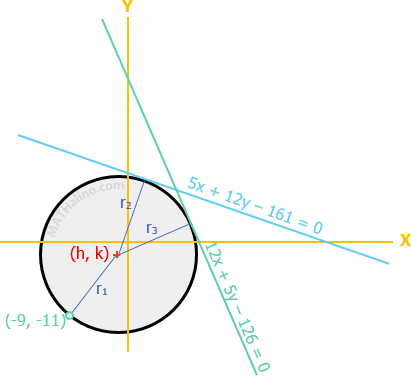# Equation of circle tangent to two lines and passing through a point

4 posts / 0 new
charlie_09Equation of circle tangent to two lines and passing through a point

Goodafternoon.,pasagot naman po ng tanong na ito,.

1. Find the equation of the circle tangent to the lines
5x + 12y - 161= 0 and 12x + 5y - 126 = 0 and passing through the point (-9,-11).

Thank you so much for your response. :-)

Jhun VertFormulas to use

Distance between two points
$d = \sqrt{(x_2 - x_1)^2 + (y_2 - y_1)^2}$

Distance from a line to a point
$d = \dfrac{ax_1 + by_1 + c}{\pm \sqrt{a^2 + b^2}}$$r_1 = \sqrt{(h + 9)^2 + (k + 11)^2}$

$r_2 = \dfrac{5h + 12k - 161}{(+)(-)\sqrt{5^2 + 12^2}} = \dfrac{5h + 12k - 161}{-13}$

$r_3 = \dfrac{12h + 5k - 126}{(+)(-)\sqrt{12^2 + 5^2}} = \dfrac{12h + 5k - 126}{-13}$

$r_2 = r_3$

$\dfrac{5h + 12k - 161}{-13} = \dfrac{12h + 5k - 126}{-13}$

$5h + 12k - 161 = 12h + 5k - 126$

$-7h + 7k - 35 = 0$

$h = k - 5$

$r_1 = r_2$

$\sqrt{(h + 9)^2 + (k + 11)^2} = \dfrac{5h + 12k - 161}{-13}$

$(h + 9)^2 + (k + 11)^2 = \dfrac{(5h + 12k - 161)^2}{169}$

$169(h + 9)^2 + 169(k + 11)^2 = (5h + 12k - 161)^2$

$169(h^2 + 18h + 81) + 169(k^2 + 22k + 121) \\ ~ ~ ~ ~ ~ = (5h)^2 + (12k)^2 + (-161)^2 + 2(5h)(12k) + 2(5h)(-161) + 2(12k)(-161)$

$(169h^2 + 3\,042h + 13\,689) + (169k^2 + 3\,718k + 20\,449) \\ ~ ~ ~ ~ ~ = 25h^2 + 144k^2 + 25\,921 + 120hk - 1\,610h - 3\,864k$

$144h^2 + 25k^2 - 120hk + 4\,652h + 7\,582k + 8\,217 = 0$

$144(k - 5)^2 + 25k^2 - 120(k - 5)k + 4\,652(k - 5) + 7\,582k + 8\,217 = 0$

$144(k^2 - 10k + 25) + 25k^2 - (120k^2 - 600k) + (4\,652k - 23\,260) + 7\,582k + 8\,217 = 0$

$144k^2 - 1\,440k + 3\,600 + 25k^2 - 120k^2 + 600k + 4\,652k - 23\,260 + 7\,582k + 8\,217 = 0$

$49k^2 + 11\,394k - 11\,443 = 0$

Now you can take it from here. Solve for k and solve for h then find the radius r. The solution is a little ugly because of the equations involved, I hope somebody will share a more beautiful approach.

Rj Campos

Ano po ang next step?

Arthur Mendiolafrom the above equation, k=1,then h=-4.solve for r from any equation for r above. then substitute the values in the equation of circle and transform to standard form.

• Mathematics inside the configured delimiters is rendered by MathJax. The default math delimiters are $$...$$ and $...$ for displayed mathematics, and $...$ and $...$ for in-line mathematics.